# Highlights

The authors propose a way to bootstrap Actor-Critic (AC) Reinforcement Learning (RL) algorithms from expert demonstrations by taking DDPG as an example.

# Introduction

AC-style RL algorithms work by having an actor $$\pi$$ act in the environment and a critic $$Q$$ output how well the actor is doing. What the critic is actually outputting is the value function $$V^\pi(s_t) = E_{a_t,s_{t+1},..}[\sum_{\tau=0}^{\infty}\gamma^\tau r(s_{t+\tau})]$$ which is the discounted reward at $$s_t$$. Let $$\eta(\pi) = E_{s_0,a_0,..}[\sum_{t=0}^{\infty}\gamma^\tau r(s_{t})]$$ be the expected sum of discounted reward for a specific policy and $$A^\pi(s_t, a_t) = Q^\pi(s_t, a_t) - V^\pi(s_t)$$ be the advantage function, telling you how good the reward from your action is compared to the expected reward at the current state.

# Methods

Let’s say we have an expert policy $$\pi^*$$ that performs better than our policy, which we can define with

$\eta(\pi^*) \geq \eta(\pi)$

which we will call constraint $$(1)$$

The authors then introduce the following theorem and “prove it”: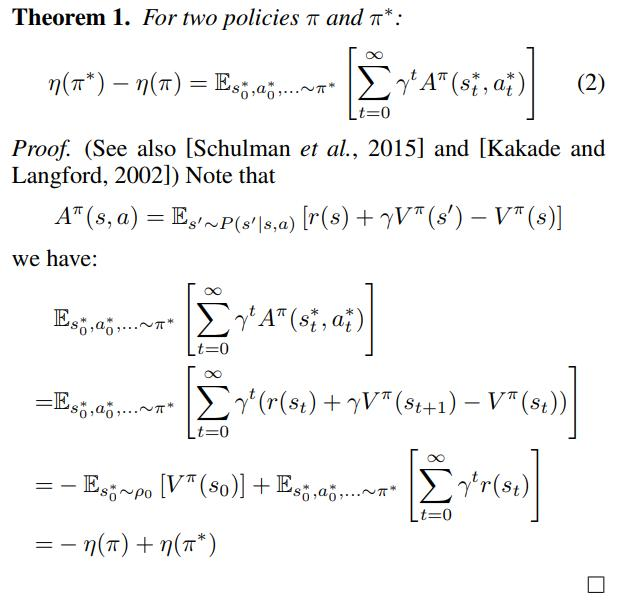which gives the constraint $$(3)$$

$E_{s_0^*,a_0^*,..~\pi} [\sum_{t=0}^{\infty}\gamma^t A^\pi (s_t^*,a_t^*)] \geq 0$

which basically says that the expert always acts better or as good as expected.

The authors then give the following gradients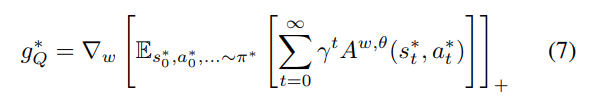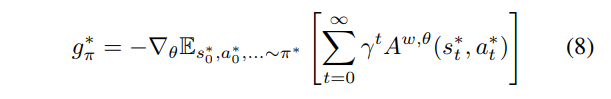where $$w, \theta$$ are the parameters for the critic and actor networks. We can then combine them to the standard DDPG gradients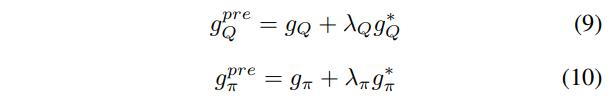TLDR: Calculate the advantage of the expert policy. Multiply the advantage to the gradients of the weights of the critic. Add it to the gradient from the raw algorithm.

## Data

The authors tested their method on the HalfCheetah, Hopper, and Walker2d OpenAI Gym environments.

# Results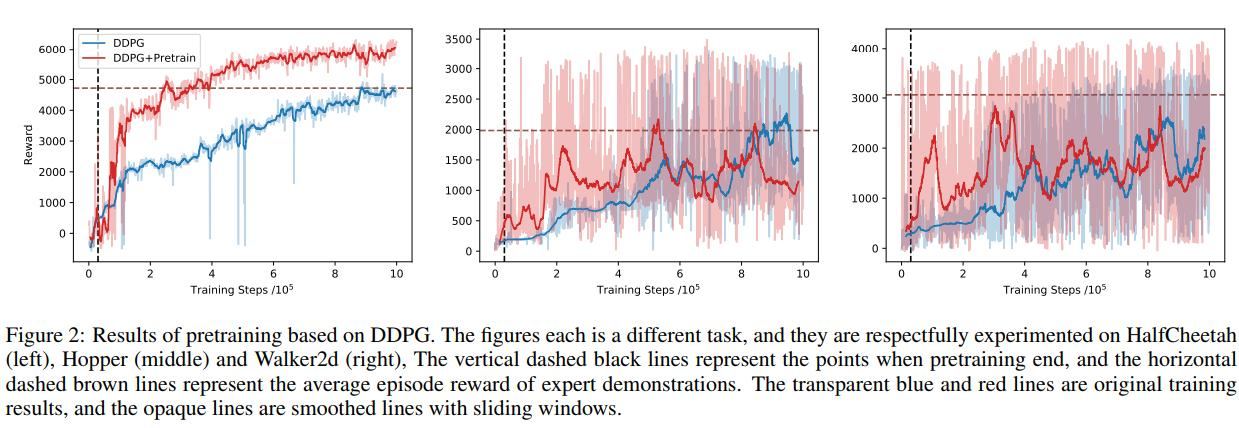# Conclusions

Pretraining Actor-Critic algorithms with expert demonstrations seem to improve performance from cold-started algorithms.

# Remarks

In fact, it doesn’t really seem to improve that much in the long run, the “theorem” is quite sketchy and I’m not sure I really follow their math.